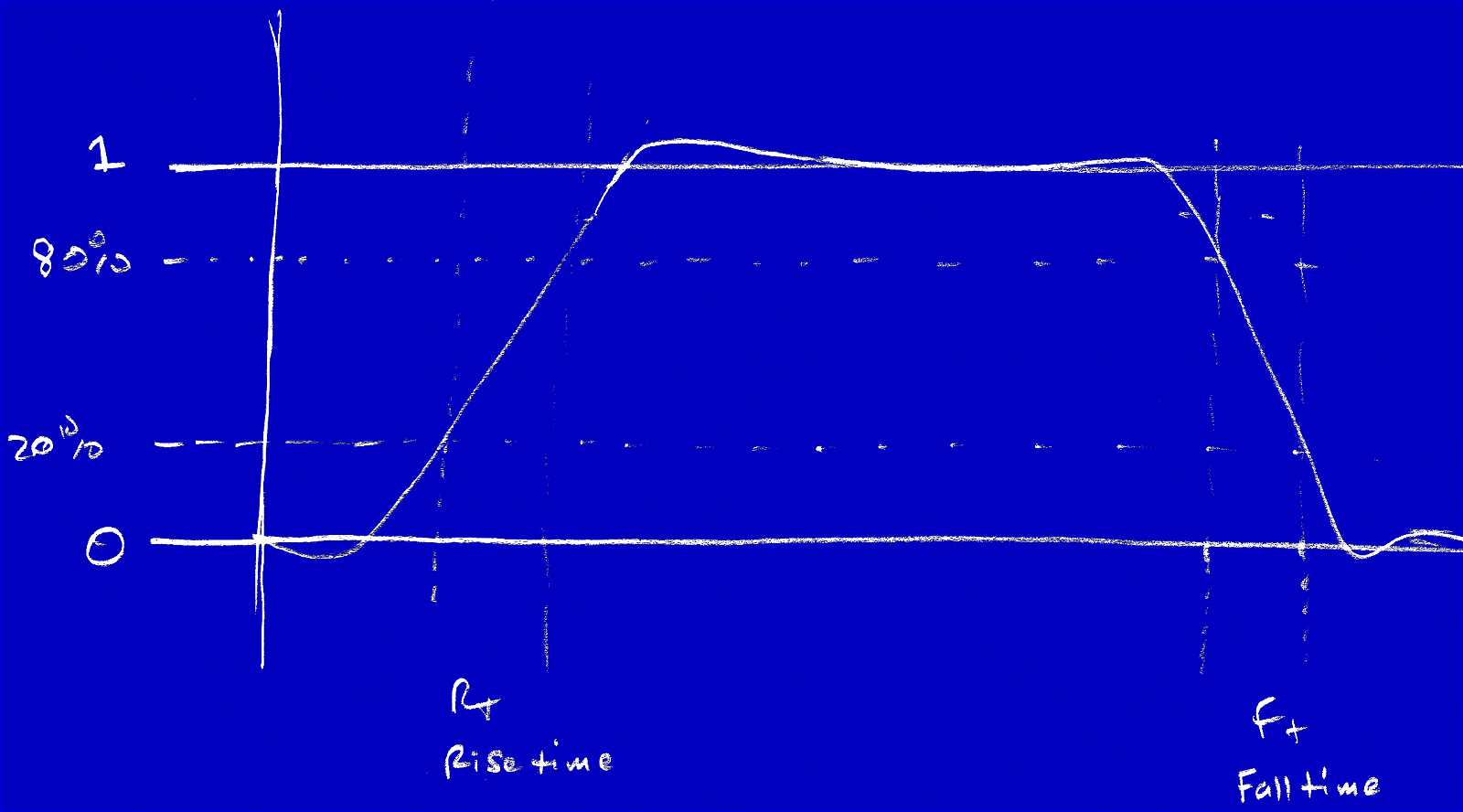### Digital Electronics: Timing Diagrams

Timing Diagrams are graphs of digital signals as a function of time.Square Wave featuring Rise-Time and Fall-Time.

Using the 50% Mark to measure the delays:• In the above image, X-Axis: Time, Y-Axis: Voltage or the Signal.
• For example, let us ask ourselves what is the delay from S1 to S2?
• So, the frame of reference, in general, is the 50% mark of the rising-edge or the falling-edge to measure the time difference Δt = t2 - t1.
• Have a common frame-of-reference.

Conventions (Shown Below):*Different companies have a slightly different timing-diagram convention.
• Tri-State means the impedance is high (using Mega-ohms values).

Asynchronous and Synchronous Timing Diagrams:Asynchronous Timing Diagram.
• Asynchronous TDs use combinatorial logic like Gates and Circuits without a clock. Output (Z) showing the propagation delay Tpd.Synchronous Timing Diagram.

• Devices and ICs like Microcontrollers, Microprocessors, Flip-Flops (single-bit memory circuits), RAMs, Memories, and clocked-circuits in general, are synchronous when a clock is used.
• Flip-Flops: T = Toggle, D = Data, SR = Set-Reset, etc are like imagine a box with gates inside it, which provide a single-bit memory.
• Example: D-Type FF => Gets the input (D) memorizes it and gives it to Q as per the sampling and it is a single bit memory (meaning, 1 FF has 1-Bit, 8 FFs connected would have 8-Bits).An example timing diagram of a D Flip-Flop shown below or above (Synchronous Timing Diagram).*The timings get violated if the Setup-Time or Hold-Time gets violated which causes the meta-stability condition. Also, generally speaking, there are around 200s of timing parameters that mean different things depending upon the kind of IC.

So, let us see a Static Memory / SRAM timing diagram (shown below):• In this, Read is HIGH and Write is LOW (refer Rd/Wr signal above).
• Other signals are: /CS = Chip Select, Data, Address (which could be either 0 or 1).
*Static memories aren't cheap.

1.Thanks for sharing the best information and suggestions, If you are looking for the best NDA0200, then TotalMedtech. Highly energetic blog, I’d love to find out some additional information.

2.I read the above article and I got some knowledge from your article. It's actually great and useful data for us. Thanks for share it. electronics parts distributor Florida

3.I am attracted by the presentation of this article about eaton easy e4. It is a genuinely gainful article for us. Keep posting, Thank you.